Chris Bell 'A business that makes nothing but money is a poor business.'
- Henry Ford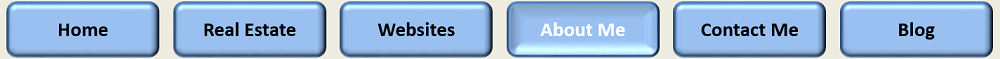Token | Discord | Videos | Sitemap

SNHU - IT-370 Web Server Design
Written by: Chris Bell - March, 2014

# Having Fun with Arithmetic Operators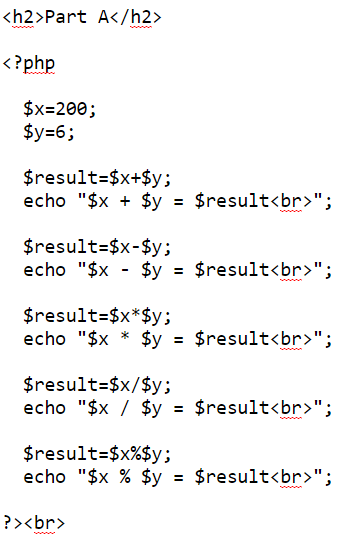## Part A

The code to the left is in action below. Try to follow the code along with the answers together below.

200 + 6 = 206
200 - 6 = 194
200 * 6 = 1200
200 / 6 = 33.333333333333
200 % 6 = 2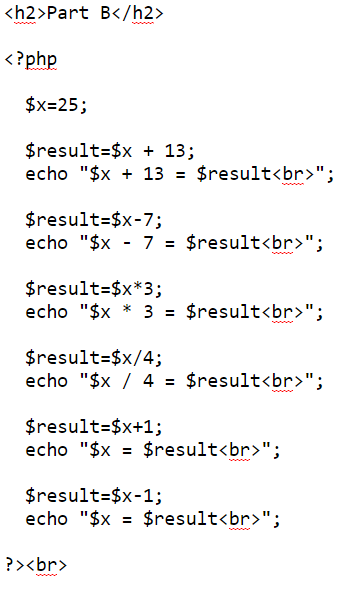## Part B

The code to the left is in action below. Try to follow the code along with the answers together below.

25 + 13 = 38
25 - 7 = 18
25 * 3 = 75
25 / 4 = 6.25
25 = 26
25 = 24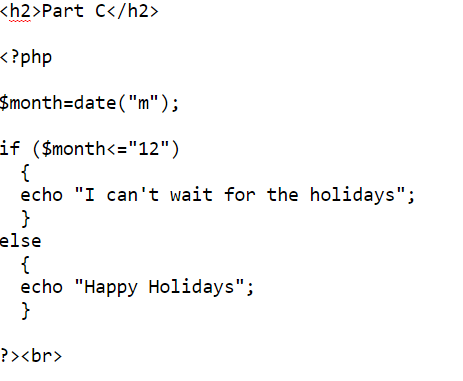## Part C

The code to the left is in action below. Try to follow the code along with the answers together below.

I can't wait for the holidays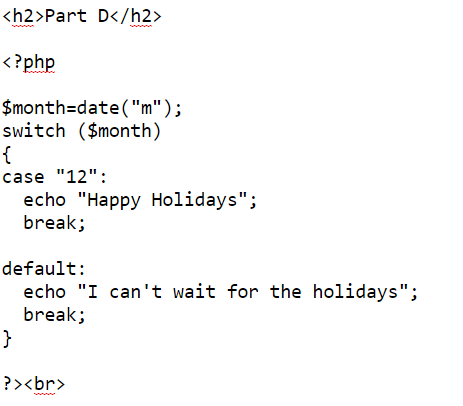## Part D

The code to the left is in action below. Try to follow the code along with the answers together below.

I can't wait for the holidays## Part E

The code to the left is in action below. Try to follow the code along with the answers together below.

1.

abc
abc
abc
abc
abc
abc
abc
abc
abc
abc

2.

xyz
xyz
xyz
xyz
xyz
xyz
xyz
xyz
xyz
xyz